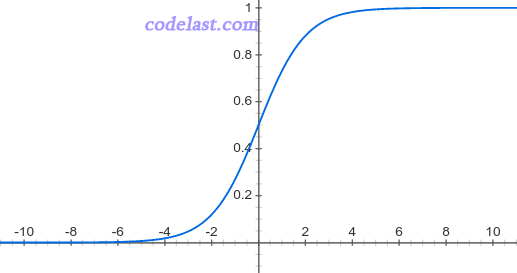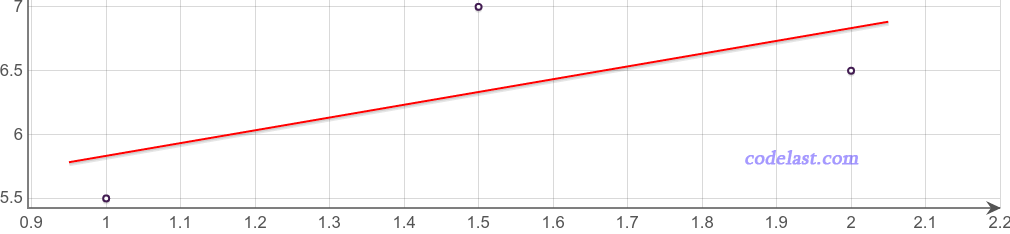Logistic Regression（或Logit Regression），即逻辑回归，简记为LR，是机器学习领域的一种极为常用的算法／方法／模型。

Logistic Regression是一种分类算法。分类，也就是把一个群体（或问题，或数据）分为几个类别，例如，男/女/人妖；爱她的人/不爱她的人；今天会下雨/今天不会下雨。
Logistic Regression最常用于处理“二分类”问题，也就是说分类只有两个，像“爱她的人/不爱她的人”就是二分类，而“男/女/人妖”就不是二分类。当然，Logistic Regression也可以用于处理多分类问题，即所谓的“多分类逻辑回归”（Multiclass Logistic Regression），但本文并不涉及这个方面。

Logistic Regression在现实世界中非常有用。例如，可以用它来判断一个用户是否会点击一个广告（会点击／不会点击），可以用Logistic Regression来判断两类人是否会相爱（会相爱／不会相爱），等等。

(1, 5.5)
(1.5, 7)
(2, 6.5)

Logistic Regression也是类似，我们有一个函数 $y = f(X)$ ，里面包含若干个未知参数 ${\theta _0},{\theta _1},{\theta _2}, \cdots ,{\theta _n}$

$f(X) = \frac{1}{{1 + {e^{ - {\theta ^T}X}}}}{\rm{ }}$ ……（1）

$s = \sum\limits_{i = 0}^n {{\theta _i}{x_i}} = {\theta _0}{x_0} + {\theta _1}{x_1} + \cdots + {\theta _n}{x_n} = {\theta ^T}X$

$P(t) = \frac{1}{{1 + {e^{ - t}}}}$$y' = \left\{ {\begin{array}{*{20}{c}}{0,f(X) > 0.5}\\{1,f(X) \le 0.5}\end{array}} \right.$

(1, 5.5)
(1.5, 7)
(2, 6.5)• 损失函数Loss Function代价函数Cost Function

WiKi的定义：
In mathematical optimization, statistics, decision theory and machine learning, a loss function or cost function is a function that maps an event or values of one or more variables onto a real number intuitively representing some "cost" associated with the event. An optimization problem seeks to minimize a loss function.

The loss function quantifies the amount by which the prediction deviates from the actual values.

（1）0-1损失函数：可用于分类问题，即该函数用于衡量分类错误的数量，但由于此损失函数是非凸（non-convex）的，因此在做最优化计算时，难以求解，所以，正因为如此，0-1损失函数不是那么“实用”（如果这句话有误，请指正）。
（2）平方损失函数（Square Loss）：常用于线性回归（Linear Regression）。
（3）对数损失（Log Loss）函数：常用于其模型输出每一类概率的分类器（classifier），例如逻辑回归。
（4）Hinge损失函数：常用于SVM（Support Vector Machine，支持向量机，一种机器学习算法）。中文名叫“合页损失函数”，因为hinge有“合页”之意。这个翻译虽然直白，但是你会发现，99％的文章都不会用它的中文名来称呼它，而是用“Hinge损失”之类的说法。

$L = - \frac{1}{n}\sum\limits_{i = 1}^n {[{y_i}\log ({{\hat y}_i}) + (1 - {y_i})\log (1 - {{\hat y}_i})]}$ ......（2）

$L = - \frac{1}{N}\sum\limits_{i = 1}^n {\left[ {{y_i}\log \left( {\frac{1}{{1 + {e^{ - {\theta ^T}{X_i}}}}}} \right) + (1 - {y_i})\log \left( {1 - \frac{1}{{1 + {e^{ - {\theta ^T}{X_i}}}}}} \right)} \right]}$

$L(\theta ) = - \frac{1}{N}\sum\limits_{i = 1}^n {\left[ {{y_i}\log \left( {\frac{1}{{1 + {e^{ - {\theta ^T}{X_i}}}}}} \right) + (1 - {y_i})\log \left( {1 - \frac{1}{{1 + {e^{ - {\theta ^T}{X_i}}}}}} \right)} \right]}$

• 求解方法

[原创] 用人话解释机器学习中的Logistic Regression（逻辑回归）

### 15 thoughts on “[原创] 用人话解释机器学习中的Logistic Regression（逻辑回归）”

• 2019 年 03 月 20 日 at 18:36

非常棒的讲解，让人豁然开朗

• 2019 年 03 月 05 日 at 01:54

非常感谢博主，没有数学基础的小白终于搞懂了！

• 2018 年 11 月 09 日 at 07:39

非常好 很感谢

• 2018 年 06 月 05 日 at 16:28

博主讲的很清晰易懂啊 之前对对数损失函数和交叉熵有疑惑 现在懂了

• 2018 年 04 月 17 日 at 23:32

Excellent 1!! 希望能看到更多机械学习的文章

• 2018 年 03 月 10 日 at 17:57

收获太多，感谢作者分享

• 2017 年 12 月 01 日 at 01:20

人話 讓人類更好理解
感謝作者!

• 2017 年 08 月 30 日 at 09:05

万谢，解答了我的疑惑，博主加油哦

• 2017 年 03 月 24 日 at 20:23

看了你的评论我对我来说就是醍醐灌顶 谢谢！ 很想认识你

• 2017 年 01 月 17 日 at 22:51

您寫得太好了, 讓我心領神會。有個問題想再請教一下，關於θ訓練出來之後帶入式子1, 是要用什麼門檻值來決定是屬於第1類還是屬於2類呢? 還是通常都使用0.5 呢?

• 2017 年 01 月 19 日 at 15:54

这个阈值是根据业务的实际情况来设置／调整的，并不是个固定值，需要根据数据来分析。

• 2016 年 09 月 25 日 at 11:40

你的答案就是我想要的